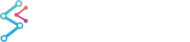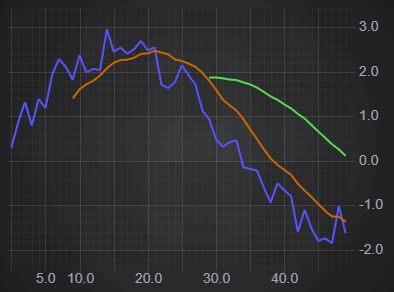﻿ Moving Average Filter | JavaScript Chart DocumentationSciChart.js JavaScript 2D Charts API > 2D Chart Types > Data Filters (Transforms) API > Moving Average Filter
Moving Average Filter

The XyMovingAverageFilter performs a simple moving average with a specified period length, resulting in a smoothed waveform derived from your data.

## Applying a Moving Average to Chart Data

To calculate a moving average and apply to a chart, use the following code.

```import { SciChartSurface } from 'scichart/Charting/Visuals/SciChartSurface';
import { NumericAxis } from 'scichart/Charting/Visuals/Axis/NumericAxis';
import { XyDataSeries } from 'scichart/Charting/Model/XyDataSeries';
import { FastLineRenderableSeries } from 'scichart/Charting/Visuals/RenderableSeries/FastLineRenderableSeries';
import { NumberRange } from 'scichart/Core/NumberRange';
import { XyMovingAverageFilter } from 'scichart/Charting/Model/Filters/XyMovingAverageFilter';
...
const { sciChartSurface, wasmContext } = await SciChartSurface.create('scichart-div-id-4');
sciChartSurface.xAxes.add(new NumericAxis(wasmContext, { growBy: new NumberRange(0.1, 0.1) }));
sciChartSurface.yAxes.add(new NumericAxis(wasmContext, { growBy: new NumberRange(0.01, 0.01), autoRange: EAutoRange.Always }));
// A function to get some data - sinewave plus a randm factor
const getData = (start, count) => {
let xValues = [];
let yValues = [];
for (let i = start; i < start + count; i++) {
xValues.push(i);
yValues.push(2 * Math.sin(i/10) + Math.random());
}
return { xValues, yValues };
};
// Original Data
const dataSeries = new XyDataSeries(wasmContext, getData(0, 100));
const originalLine = new FastLineRenderableSeries(wasmContext, { dataSeries, stroke: "#5555ff", strokeThickness: 3 });
// Create the filter, passing in the original series
const movingAverage = new XyMovingAverageFilter(dataSeries, { length: 10 });
const filteredLine = new FastLineRenderableSeries(wasmContext, { dataSeries: movingAverage, stroke: "#cc6600", strokeThickness: 3 });
// Another filter using the same original data, but different length
const movingAverage30 = new XyMovingAverageFilter(dataSeries, { length: 30});
const filteredLine30 = new FastLineRenderableSeries(wasmContext, { dataSeries: movingAverage30, stroke: "#55dd55", strokeThickness: 3 });
```

This results in the following output:## Updating Moving Averages Dynamically

When the underlying data updates, the filter automatically updates. There is no need to recalculate your filter: SciChart.js does this for you!

Where possible, only the changed points are recalculated. In addition, updating the parameters of the filter, in this case the length, will also recalulate the filter and redraw.

If we add the following to the above example:

```// Add some additional data every 100ms
const updateFunc = () => {
if (dataSeries.count() < 300) {
const { xValues, yValues } = getData(dataSeries.count(), 10);
dataSeries.appendRange(xValues, yValues);
setTimeout(updateFunc, 100);
}
};
// Start the update
setTimeout(updateFunc, 1000);
```

We get this output.